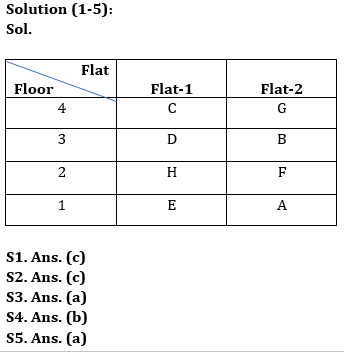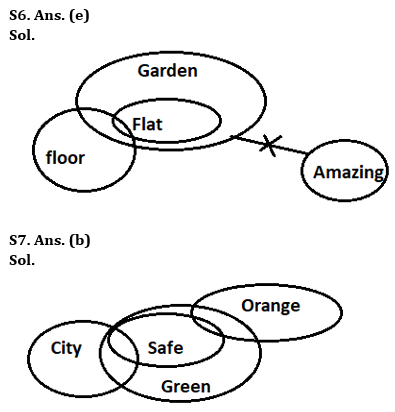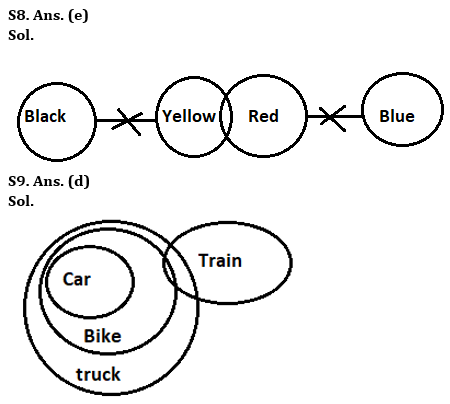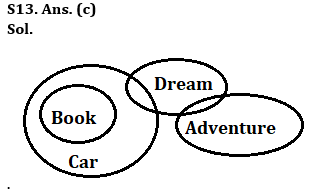Latest Banking jobs   »

# Reasoning Ability Quiz For Bank Foundation 2023 -18th April

Directions (1-5): Study the information carefully and answer the questions given below.
Eight persons A, B, C, D, E, F, G, and H live on four different floors of a building but not necessarily in the same order. Ground floor is numbered as 1, floor above it is numbered as 2 and so on till topmost floor which is numbered as 4. There are two flats on each floor- flat-1 and flat-2 from west to east such that flat 1 is west of flat 2. Flat-1 of second floor is exactly above flat-1 of first floor and exactly below the flat-1 of third floor, other flats are placed in the same way.
H does not live at an odd number floor. B’s flat is south of G’s flat and north-east of H’s flat. D lives at an odd number floor and on an odd number flat. Two floors gap is there between A’s flat and C’s flat. C’s flat is north-west of A’s flat. E does not live on the same floor as H. F does not live just below the floor of E.

Q1. Who among the following persons lives just above F on the same flat as F?
(a) C
(b) A
(c) B
(d) D
(e) None of these

Q2. Who among the following persons lives east of H?
(a) G
(b) B
(c) F
(d) A
(e) None of these

Q3. How many floors are there between E’s floor and G’s floor?
(a) Two
(b) One
(c) Either Two or One
(d) Both lives at the same floor
(e) None of these

Q4. Who among the following person lives at Flat-1 of floor-4?
(a) A
(b) C
(c) B
(d) G
(e) None of these

Q5. Who among the following persons lives in flat-2 of floor-1?
(a) A
(b) B
(c) E
(d) F
(e) None of these

Directions (6-9): In each of the questions below some statements are given followed by some Conclusions. You have to take the given statements to be true even, if they seem to be at variance from commonly known facts. Read all the conclusions and then decide which of the given conclusions logically follows from the given statements disregarding commonly known facts.
(a) If only conclusion I follows.
(b) If only conclusion II follows.
(c) If either conclusion I or II follows.
(d) If neither conclusion I nor II follows.
(e) If both conclusions I and II follow.

Q6. Statements:
Some floor are flat
All flat are garden
No garden is amazing
Conclusions:
I: Some floor are not amazing
II: No Flat is amazing

Q7. Statements:
Only a few city are safe
All safe are green
Some safe are orange
Conclusions:
I: Some city are orange
II: Some orange are green

Q8. Statements:
Some yellow are red
No red is blue
No yellow is black
Conclusions:
I. Some red are not black
II. Some yellow are not blue

Q9. Statements:
All car are bike
All bike is truck
Some bike are train
Conclusions:
I. All bike are car
II. All truck are train

Directions (10-12): In these questions, relationships between different elements are shown in the statements. These statements are followed by two conclusions. Give answer
(a) if only conclusion I follows
(b) if only conclusion II follows
(c) if either conclusion I or conclusion II follows
(d) if neither conclusion I nor conclusion II follows
(e) if both conclusions I and II follow

Q10. Statement: W>R<T≤Y>C=D
Conclusions: I. C>R II.T≤D

Q11. Statement: A≥B>C=E≤D=F<G
Conclusions: I. G>C II.E≤A

Q12. Statements: H>S>U=Z>N≤J=Q>M
Conclusion I: Z<H II: Q>U

Direction (13): In each of the questions below some statements are given followed by two conclusions. You have to take the given statements to be true even if they seem to be at variance with commonly known facts. Read all the conclusions and then decide which of the given conclusions logically follows from the given statements disregarding commonly known facts.
(a) If only conclusion I follows.
(b) If only conclusion II follows.
(c) If either conclusion I or II follows.
(d) If neither conclusion I nor II follows.
(e) If both conclusions I and II follow.

Q13. Statements:
All book are Car
Some Car is dream
Conclusion
I: Some Dream are book
II: No book is dream

Direction (14-15): In the following questions assuming the given statement to be true, find which of the conclusion(s) among given conclusions is/are definitely true and then give your answers accordingly.
(a) Only conclusion I is true
(b) Only conclusion II is true
(c) Either conclusion I or II is true
(d) Neither conclusion I nor II is true
(e) Both conclusions I and II are true

Q14. Statements: A > G ≥ F = H < K ≤ S > R
Conclusions: I. A > H II. F ≤ S

Q15. Statements: D ≥ P > O < Y ≥ T ≤ E ≤ R
Conclusions: I. P > Y II. O < E

SolutionsSolution: (10-12):
S10. Ans.(d)
Sol. I. C>R (false) II.T≤D (false)

S11. Ans.(a)
Sol. I. G>C (true) II.E≤A (false)

S12. Ans. (a)
Sol. I: Z<H (true) II: Q>U(false)Solution (14-15):
S14. Ans. (a)
Sol. I. A > H(true) II. F ≤ S(false)
S15. Ans. (d)
Sol. I. P > Y(false) II. O < E(false)## FAQs

### What is the selection process of the Bank Clerk?

The selection process of the Bank Clerk is Prelims & Mains.

#### Congratulations!Union Budget 2023-24: Free PDF